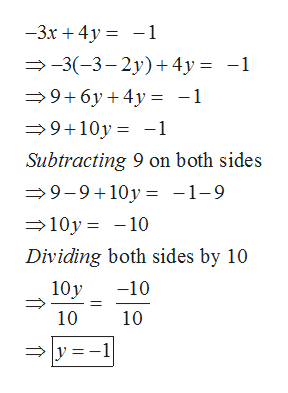# Solve the system by substitution -3x+4y= -14x+8y= -12

Question
16 views

Solve the system by substitution

-3x+4y= -1

4x+8y= -12

check_circle

Step 1

We have equations :-3x+4y= -1, 4x+8y= -12.

Let us isolate equation (2) for x first.

Dividing equation (2) by 4 .

Then subtracting 2y on both sides.

Step 2

Substituting x=-3-2y in first equation -3x+4y=-1 and solve for y.

We got y=-1.help_outlineImage Transcriptionclose-3r4y 1 -3(-3-2y) +4y -1 9 6y 4y -1 9 10y-1 Subtracting 9 on both sides 9-9 10y= -1-9 10y 10 Dividing both sides by 10 10y -10 10 10 y =1 fullscreen
Step 3

Plugging y=-1 in x=-3-2...

### Want to see the full answer?

See Solution

#### Want to see this answer and more?

Solutions are written by subject experts who are available 24/7. Questions are typically answered within 1 hour.*

See Solution
*Response times may vary by subject and question.
Tagged in

### Algebra